# Geometry and Measure Worksheets

Here you will find all our GCSE geometry and measure worksheets for secondary schools.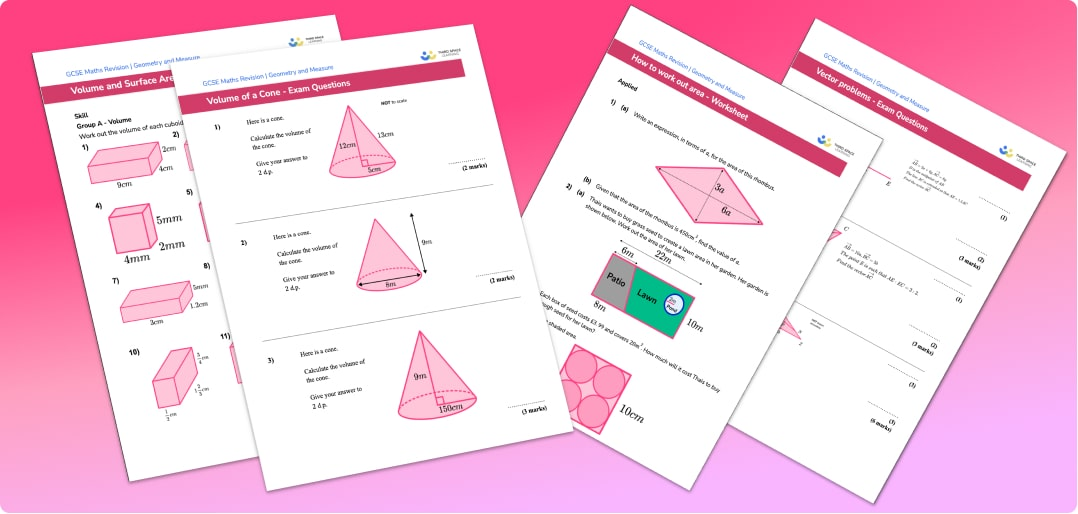What can you expect from the geometry and measure worksheets?

The free geometry and measure worksheets comprise functional and applied reasoning questions, practice questions, exam style questions and word problems.

These printable maths worksheets are suitable for use as part of GCSE maths revision for all major exam boards including Edexcel, AQA and OCR.

Designed to be used within our step-by-step GCSE maths revision lessons.

Mark scheme and answers included for every free geometry and measure worksheet.

Filter by:

Algebra

Geometry

Number

Probability

Ratio and Proportion

Statistics

Mixed

Algebraic expressions

Simplifying expressions

Expanding brackets

Factorising

Equations

Solving equations

Rearranging equations

Simultaneous equations

Maths formulas

Interpreting graphs

Types of graphs

Inequalities

Sequences

Functions in algebra

Laws of indices

Mathematical proof

2D Shapes

Polygons

Symmetry

Area

How to work out perimeter

Circles, sectors and arcs

Angles

Angle rules

Angles in polygons

Angles in parallel lines

3D shapes

How to calculate volume

Prism

Cuboid

Triangular prism

Sphere

Hemisphere shape

Cone

Cylinder

Pyramid

Pythagoras theorem

Trigonometry

Circle theorems

Vectors

Loci And Construction

Transformations

Congruence and similarity

Types of Graphs

Interpreting Graphs

Arithmetic

Rounding numbers

Factors, multiples and primes

Negative numbers

Fractions, decimals and percentages

Fractions

Decimals

Percentages

Comparing fractions decimals and percentages

Powers and roots

Standard form

Simple interest and compound interest

Types of numbers

Surds

Describing probability

Probability distribution

How to calculate probability

Combined events probability

Ratio

Proportion

Compound measures

Scale maths

Units of measurement

Conversion graphs

Rate of change

Types of data

Mean, median, mode

Representing data

Frequency table

Cumulative frequency

Histogram

Types of sampling methods

Clear filter

## How to use the geometry and measure worksheets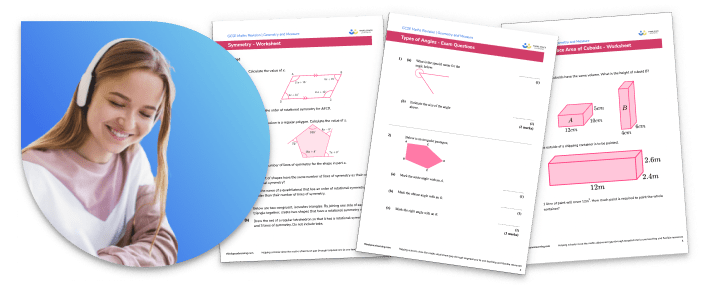Students will explore key geometry skills in order to answer questions on classifying 2d shapes (polygons) like quadrilaterals (such as rectangles and parallelograms), and triangles (such as equilateral or isosceles).

Students will look at whether shapes are congruent by examining their sides and vertices, and by measuring angles.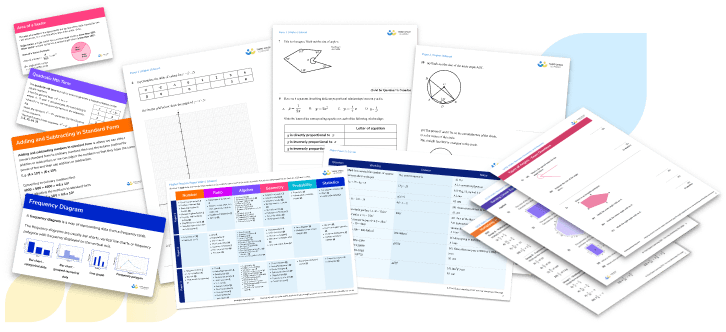"Questions test skills such as area of a triangle and other shapes as well as surface area of 3D shapes including prisms by using nets.

Pythagoras theorem and trigonometry questions build on this to work out missing angles and sides in right angled triangles, and the sine and cosine rules are used for other triangles. Trigonometric graphs can be used to support this. While drawing graphs, skills developed while learning about constructions and how to use a protractor are vital.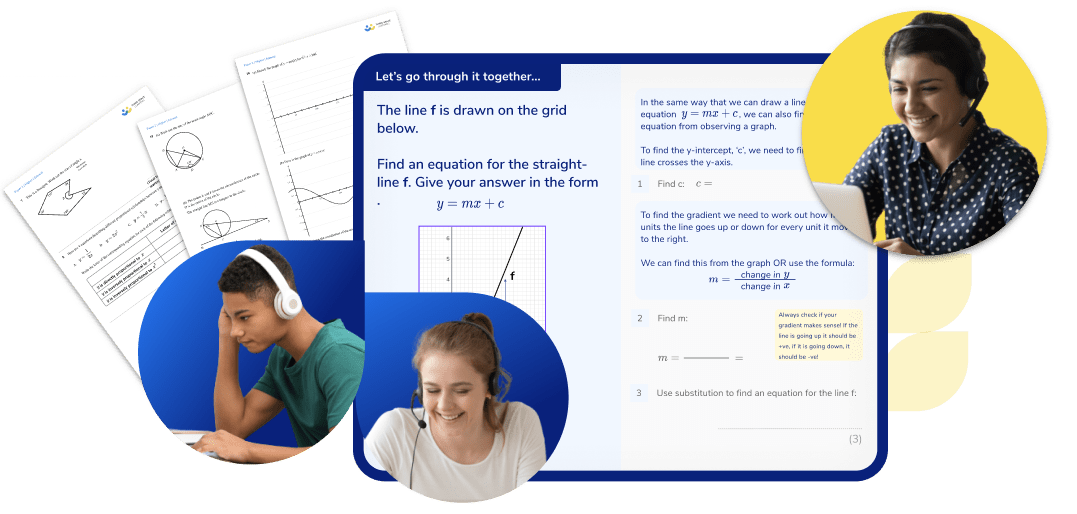You can find further GCSE maths resources within these step by step Geometry and Measure lessons.

For any students struggling with Geometry and Measure, our GCSE maths revision programme of online tuition provides the one to one personalised support they need to develop understanding and make rapid progress.

## Can't find what you're looking for?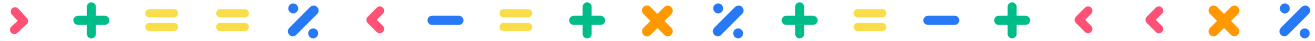## Teaching support from the UK’s largest provider of in-school maths tuition

At Third Space Learning, we’re on a mission to help teachers like you shape your students into confident, able mathematicians.

Request a quote